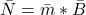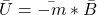## A permanent magnet has a magnetic dipole moment of 0.160 A · m^2. The magnet is in the presence of an external uniform magnetic field (provi

Question

A permanent magnet has a magnetic dipole moment of 0.160 A · m^2. The magnet is in the presence of an external uniform magnetic field (provided by current-carrying coils) with a magnitude of 0.0800 T, which makes an angle of 35.0° with the orientation of the permanent magnet.

a. What is the magnitude of the torque (in N · m) on the permanent magnet?
b. What is the potential energy (in J) of the system consisting of the permanent magnet and the magnetic field provided by the coils?

in progress 0
7 months 2021-07-15T01:51:17+00:00 1 Answers 12 views 0

## Answers ( )

the magnitude of the torque  on the permanent magnet = 7.34×10⁻³ Nm

the potential energy (in J) of the system consisting of the permanent magnet and the magnetic field provided by the coils =  -1.0485 ×10⁻² J

Explanation:

The torque is given by :where ;

m = 0.160 A.m²

B = 0.0800 T

θ = 35°

So the magnitude of the torque N = mBsinθ

N = (0.160)(0.0800)(sin 35°)

N = 0.007341

N = 7.34×10⁻³ Nm

Hence, the magnitude of the torque  on the permanent magnet = 7.34×10⁻³ Nm

b) The potential energyU = -mBcosθ

U = (- 0.160)(0.0800)(cos 45)

U = -0.010485

U = -1.0485 ×10⁻² J

Thus, the potential energy (in J) of the system consisting of the permanent magnet and the magnetic field provided by the coils =  -1.0485 ×10⁻² J